# Lebedev-Skal'skaya transform

Skal'skaya–Lebedev transform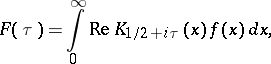(a1)

whereandis the Macdonald function. This transformation was introduced by N.N. Lebedev and I.P. Skal'skaya and investigated in connection with possible applications to certain problems in mathematical physics. It is also called the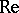-transform.

The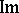-transform was initiated by them as well: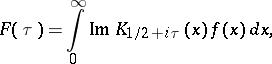(a2)

where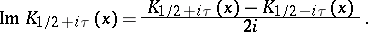Ifis an integrable function on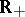with respect to the weight, i.e.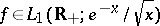, then the Lebedev–Skal'skaya transforms (a1), (a2) exist and represent bounded continuous functions on the positive half-axis which tend to zero at infinity (an analogue of the Riemann–Lebesgue lemma, cf. also Fourier series).

Let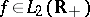. Then the Lebedev–Skal'skaya transforms (a1), (a2) converge in the mean-square sense to functions belonging to the space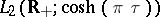and isomorphically map these two spaces onto each other. Moreover, the Parseval equality holds (see [a5])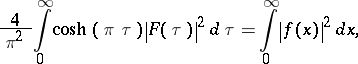as well as the inversion formulas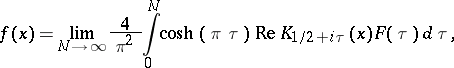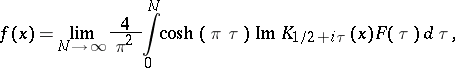for the two transforms, respectively, where the integrals are understood in the mean-square sense.

If two functions,are from the space, thendefines a convolution (cf. also Convolution of functions), for instance for the Lebedev–Skalskaya transform (a1),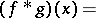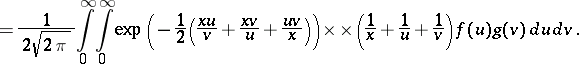The convolution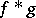belongs to the same space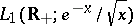and satisfies the norm estimate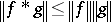in this space. The result of the action of the Lebedev–Skal'skaya transform (a1) on this convolution gives the product, where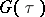is the Lebedev–Skal'skaya transform of the function.

If, moreover,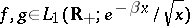,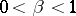, then the following integral representation of the convolution holds: